# Supported shapes of parametric cross-section¶

Note

Examples below are presented for “LCS of cross-section” set as ZYX in the sheet Project and model specification. For the other types, adjust the axis of the coordinate system of cross-section to the defined value of “LCS of cross-section” .

Pattern

Shape

Parameters [mm]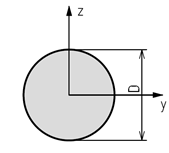Circle

D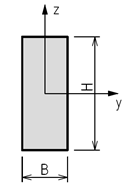Rectangle

H; B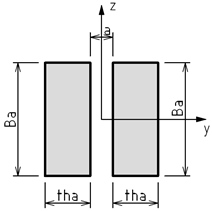Double rectangle

tha; Ba; a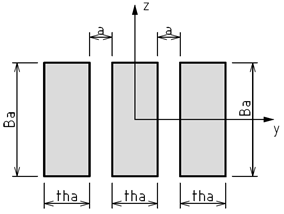Triple rectangle

tha; Ba; a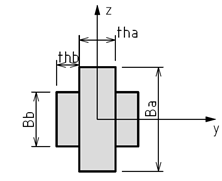Rectangle with plates

tha; Ba; thb; Bb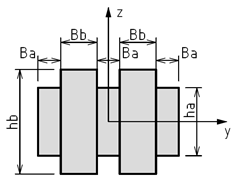Double rectangle with plates

Ba; ha; Bb; hb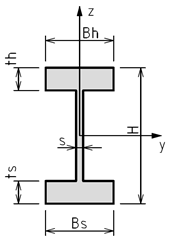I Section

H; Bh; Bs; ts; th; s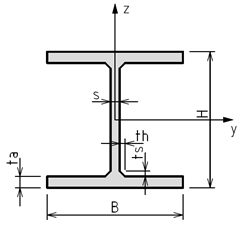I Section with haunch

H; B; ta; s; ts; th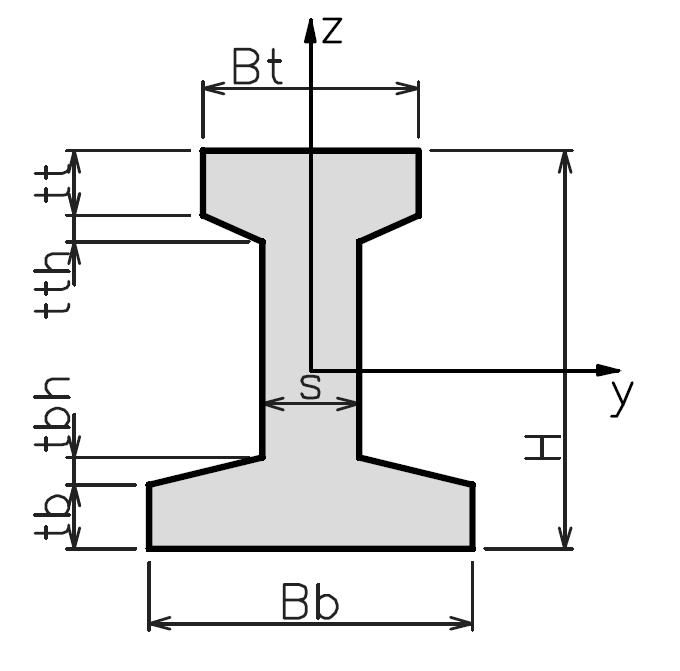I Section with haunch Asymmetric

H; Bt; tt; tth; Bb; tb; tbh; s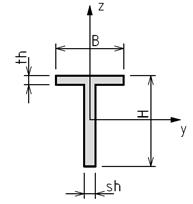T Section

H; B; th; sh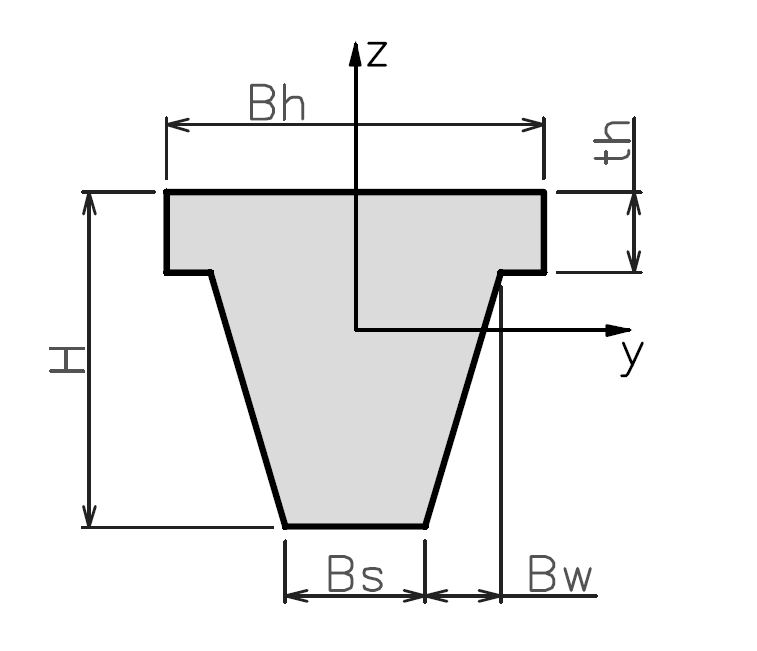T Section with haunch

H; Bh; Bs; Bw; th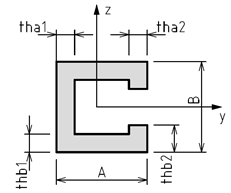C Section

A; tha1; tha2; B; thb1; thb2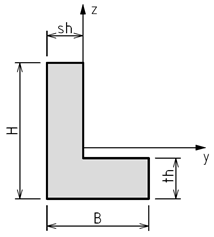L Section

H; B; th; sh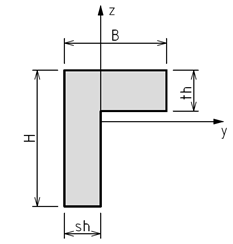L Section opposite

H; B; th; sh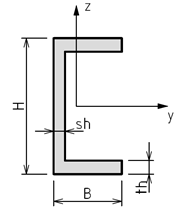U Section

H; B; th; sh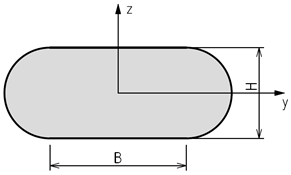Oval

H; B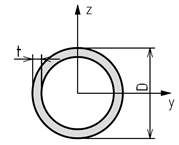Pipe

D; t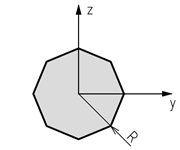Polygon

R; n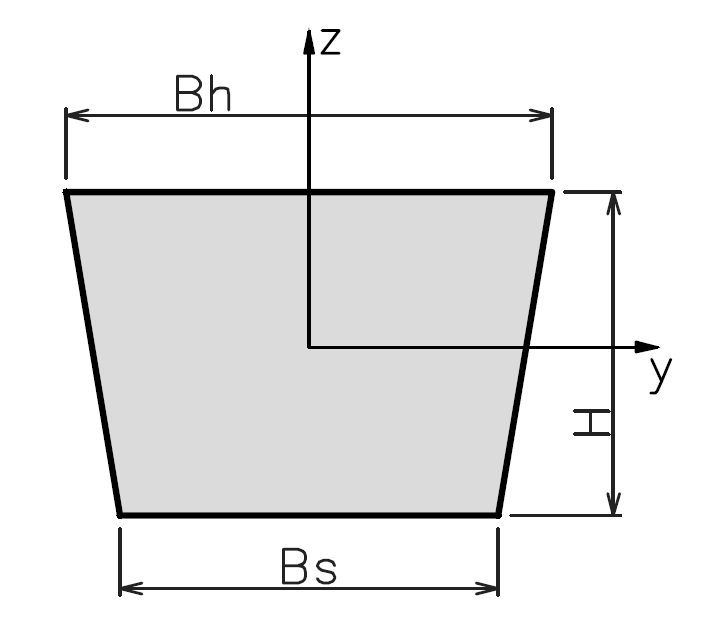Trapezoid

H; Bh; Bs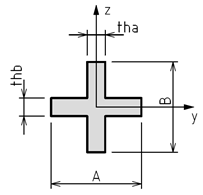X Section

A; tha; B; thb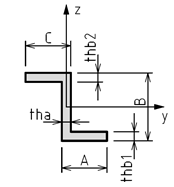Z Section

A; tha; B; thb1; thb2; C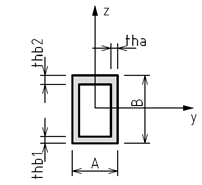Box

A; tha; B; thb1; thb2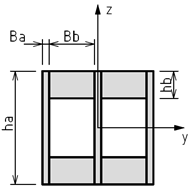Double box

Ba; ha; Bb; hb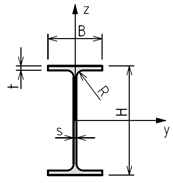I rolled

H; B; t; s; R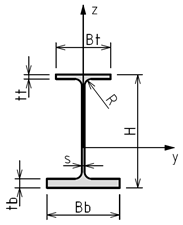I rolled asymmetric

H; s; Bt; Bb; tt; tb; R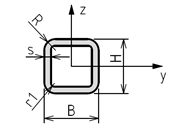Tube

H; B; s; R; r1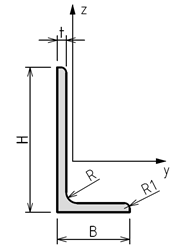Angle

H; B; t; R; R1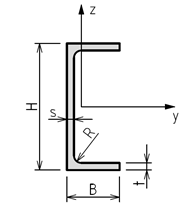Channel

H; B; t; s; R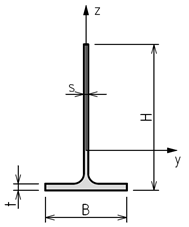T tee

H; B; t; s; R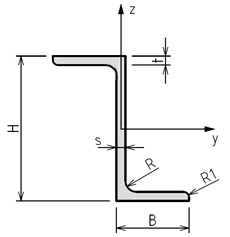Z zee

H; B; t; s; R; R1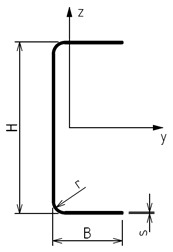Cold formed Channel

H; B; s; r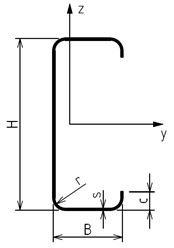Cold formed Channel with lips

H; B; s; r; c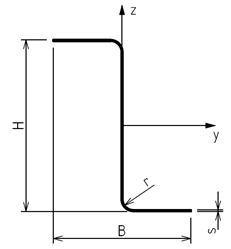Cold formed Zee

H; B; s; r